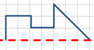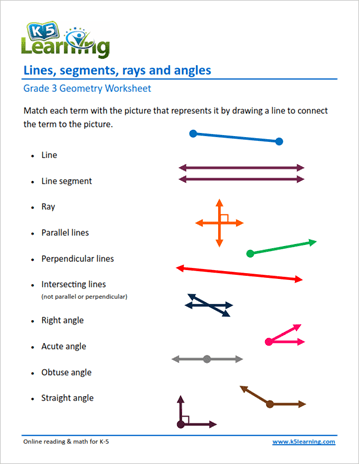# 3rd Grade Math Worksheets: Geometry

Our grade 3 geometry worksheets review two dimensional shapes, particularly circles, triangles, quadrilaterals and polygons.  We also focus on the definition and classification of lines and angles. The areas and perimeters of rectangular shapes are reviewed, as are the concepts of congruency and  symmetry.

## Basic properties of 2-D shapes

Squares, rectangles, trapezoids, parallelograms & rhombuses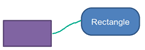A shape with 4 sides & 4 angles ....
Parallelograms
Identify quadrilaterals with 2 pairs of parallel sides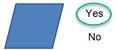Triangles
Classify triangles by sides and angles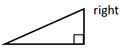Circles
Identify and label the parts of a circle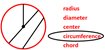Polygons
Identify regular and irregular polygons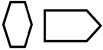Types of polygons
Classify and draw polygons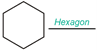## Lines and angles

Lines, segments and rays
Identify lines, segments and rays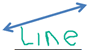Right angles
Identify right angles and compare angles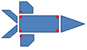Parallel and perpendicular lines
Identify parallel and perpendicular lines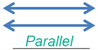Classify angles
Classify angles as straight, right, acute or obtuse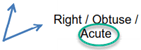Protractors
Use a protractor to draw and measure angles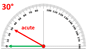Measure angles
Measure and classify angles as right, acute or obtuse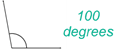Lines, segments, rays and angles
Identify and draw lines, segments, rays and angles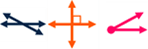## Area and perimeters

Perimeters using a rectangular grid
Find the perimeter of rectangular shapes on a grid

Areas using rectangular grids
Find the area of rectangles on a grid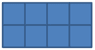Perimeters of irregular shapes
Find the perimeters of irregular shapes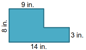Perimeters of rectangles
Find the rectangle's perimeter

Area of rectangles
Calculate the area of rectangles

Area and perimeters of rectangles
Calculate the area and perimeters of rectangles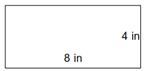## Congruency and Symmetry of 2-D shapes

Congruent shapes
Identify shapes with the same size and shape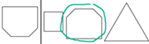Lines of symmetry
Identify and draw lines of symmetry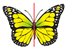Symmetrical shapes
Draw the other half of an image to form a symmetrical shape